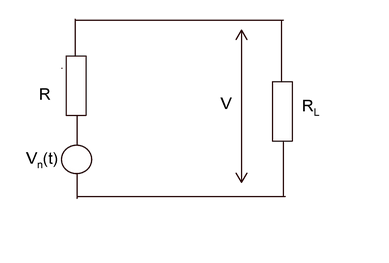# Relation between Noise voltage and bandwidth

Q8. The noise voltage (Vn)and the signal bandwidth (B) are related as

Options:

a) a) Vn is directly proportional to bandwidth
b) b) Vn is directly proportional to √bandwidth
c) c) Vn is inversely proportional to absolute temperature
d) d) Vn is inversely proportional to bandwidth

Correct Answer: b) Vn is directly proportional to √bandwidth

Explanation :

Maximum Noise power is given by Pn = V2/ RRL = R, for maximum power transfer from Vn to load resistor RL
In the above figure, according to voltage divider method,
V= Vn/2
Pn = V2/ R
= (Vn/2)2/ R
=Vn2/ 4R
Vn2= 4R Pn
Vn= √(4R Pn)
Noise power Pn= KTB
Where
K = 1.381×10-23 J/K, joules per Kelvin, the Boltzmann constant
B is the bandwidth at which the power Pn is delivered.
T noise temperature
Therefore, Vn= √(4R KTB) or Vn is directly proportional to √bandwidth.